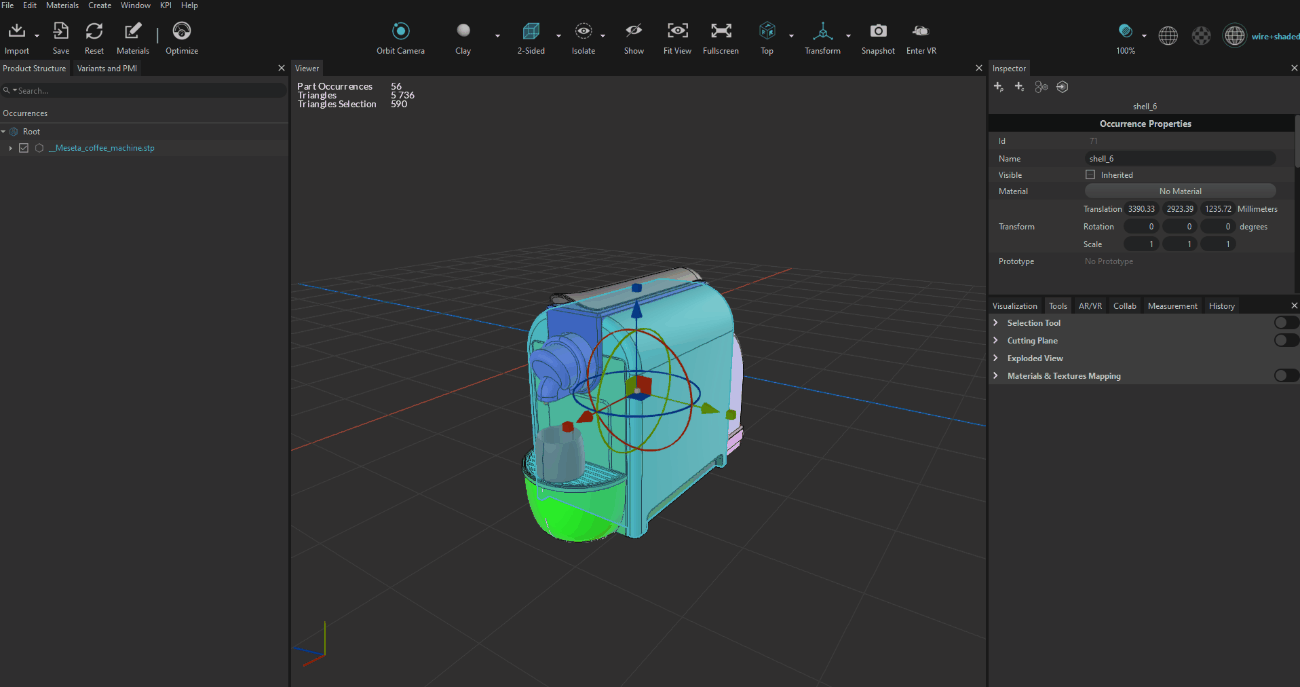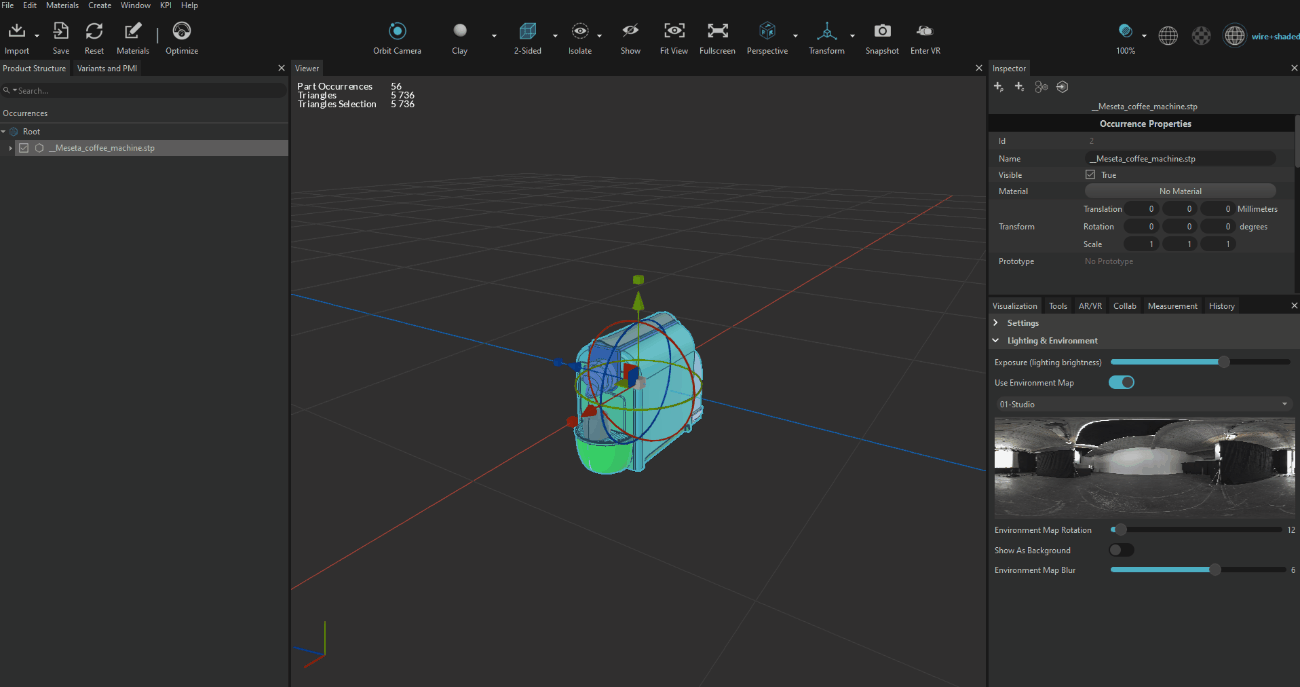You can transform an occurrence by 2 ways :

# Manipulating the Gizmo

You can either :

• Pull the triangles to operate a translation
• Grab circles and make occurrence(s) rotate
• Grab the little box to scale the occurrence

NOTE                The little white box at the center of the occurrence allows you to scale the occurrence uniformly on the 3 axis# Using the Transform Matrix

You can as well use the Transform Matrix in the inspector to put exact value to move, rotate or scale your occurrence.

Each column represents an axis (X,Y,Z).

To remember which axis were used, keep in mind that RGB = XYZ where :

• Red = X axis
• Green = Y axis
• Blue = Z axis